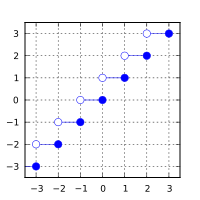/C

# ceil, ceilf, ceill

Defined in header `<math.h>`
`float       ceilf( float arg );`
(1) (since C99)
`double      ceil( double arg );`
(2)
`long double ceill( long double arg );`
(3) (since C99)
Defined in header `<tgmath.h>`
`#define ceil( arg )`
(4) (since C99)
1-3) Computes the smallest integer value not less than `arg`.
4) Type-generic macro: If `arg` has type `long double`, `ceill` is called. Otherwise, if `arg` has integer type or the type `double`, `ceil` is called. Otherwise, `ceilf` is called.

### Parameters

 arg - floating point value

### Return value

If no errors occur, the smallest integer value not less than `arg`, that is ⌈arg⌉, is returned.

Return valueArgument

### Error handling

Errors are reported as specified in math_errhandling.

If the implementation supports IEEE floating-point arithmetic (IEC 60559),

• The current rounding mode has no effect.
• If `arg` is ±∞, it is returned, unmodified
• If `arg` is ±0, it is returned, unmodified
• If arg is NaN, NaN is returned

`FE_INEXACT` may be (but isn't required to be) raised when rounding a non-integer finite value.

The largest representable floating-point values are exact integers in all standard floating-point formats, so this function never overflows on its own; however the result may overflow any integer type (including `intmax_t`), when stored in an integer variable.

This function (for double argument) behaves as if (except for the freedom to not raise `FE_INEXACT`) implemented by.

```#include <math.h>
#include <fenv.h>
#pragma STDC FENV_ACCESS ON
double ceil(double x)
{
double result;
int save_round = fegetround();
fesetround(FE_UPWARD);
result = rint(x); // or nearbyint
fesetround(save_round);
return result;
}```

### Example

```#include <math.h>
#include <stdio.h>
int main(void)
{
printf("ceil(+2.4) = %+.1f\n", ceil(2.4));
printf("ceil(-2.4) = %+.1f\n", ceil(-2.4));
printf("ceil(-0.0) = %+.1f\n", ceil(-0.0));
printf("ceil(-Inf) = %+f\n",   ceil(-INFINITY));
}```

Possible output:

```ceil(+2.4) = +3.0
ceil(-2.4) = -2.0
ceil(-0.0) = -0.0
ceil(-Inf) = -inf```
• C11 standard (ISO/IEC 9899:2011):
• 7.12.9.1 The ceil functions (p: 251)
• 7.25 Type-generic math <tgmath.h> (p: 373-375)
• F.10.6.1 The ceil functions (p: 526)
• C99 standard (ISO/IEC 9899:1999):
• 7.12.9.1 The ceil functions (p: 231-232)
• 7.22 Type-generic math <tgmath.h> (p: 335-337)
• F.9.6.1 The ceil functions (p: 462-463)
• C89/C90 standard (ISO/IEC 9899:1990):
• 4.5.6.1 The ceil function